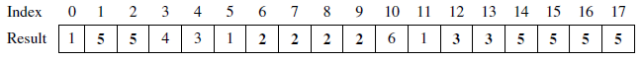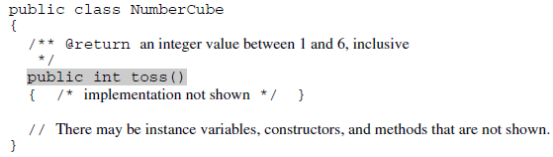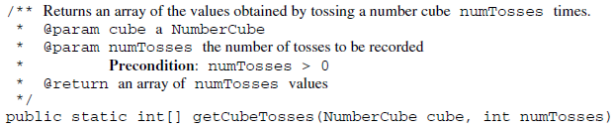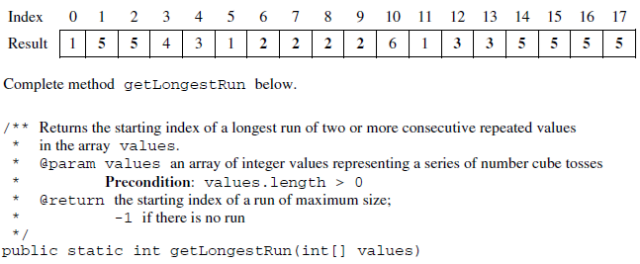# 2009 AP Computer Science A 计算机科学A真题系列之简答题免费下载

### 真题与答案下载### 2009 AP 计算机科学A简答题部分免费下载

#### 部分真题预览：

1）A statistician is studying sequences of numbers obtained by repeatedly tossing a six-sided number cube. On each side of the number cube is a single number in the range of 1 to 6, inclusive, and no number is repeated on the cube. The statistician is particularly interested in runs of numbers. A run occurs when two or more consecutive tosses of the cube produce the same value. For example, in the following sequence of cube tosses, there are runs starting at positions 1, 6, 12, and 14.The number cube is represented by the following class.You will implement a method that collects the results of several tosses of a number cube and another method that calculates the longest run found in a sequence of tosses.

1. Write the method getCubeTosses that takes a number cube and a number of tosses as parameters. The method should return an array of the values produced by tossing the number cube the given number of times.
Complete method getCubeTosses below.2. Write the method getLongestRun that takes as its parameter an array of integer values representing a series of number cube tosses. The method returns the starting index in the array of a run of maximum size. A run is defined as the repeated occurrence of the same value in two or more consecutive positions in the array.
For example, the following array contains two runs of length 4, one starting at index 6 and another starting at index 14. The method may return either of those starting indexes.
If there are no runs of any value, the method returns -1.### 2009 AP Computer Science A 计算机科学FRQ简答题完整版答案免费下载